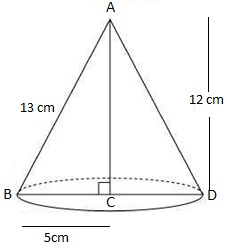Guru

# A right triangle ABC with sides 5cm, 12cm and 13cm is revolved about the side 12 cm. Find the volume of the solid so obtained. Q.7

• 0

Please give me the best way for solving the problem of class 9th ncert math of Surface areas and Volumes chapter of math of class 9th of exercise 13.7  of question no 7 what is the tricky way for solving this question A right triangle ABC with sides 5cm, 12cm and 13cm is revolved about the side 12 cm. Find the volume of the solid so obtained.

Share

1. Height (h)= 12 cm

Radius (r) = 5 cm, and

Slant height (l) = 13 cmVolume of cone, V = (1/3) πr2h

V = (1/3)×π×52×12

= 100π

Volume of the cone so formed is 100π cm3.

• 0